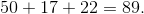ISEE Lower Level Quantitative : How to add

Example Questions

1 2 9 10 11 12 13 14 15 17 Next →

Example Question #99 : How To Add

For a project, Tim needspieces of blue paper,pieces of yellow paper, andpieces of green paper. How many pieces of paper does Tim need?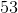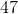Explanation:

This is an addition problem because we want to know how many total pieces of paper Tim needs all together. We can add the numbers is any order,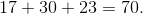Example Question #100 : How To Add

A bag of marbles haspurple marbles,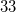black marbles, andred marbles. How many marbles are in the bag?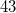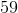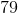Explanation:

This is an addition problem because we want to know how many total marbles are in the bag all together. We can add the numbers in any order,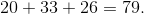Example Question #5 : Using Addition Within 100 To Solve Word Problems

At the dog park there arebrown dogs,white dogs, andblack dogs. How many dogs are at the park?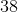Explanation:

This is an addition problem because we want to know how many total dogs are at the park all together. We can add the numbers in any order,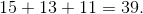Example Question #6 : Using Addition Within 100 To Solve Word Problems

At school we had a hotdog-eating contest. I atehotdogs, Mel atehotdogs, and Eric atehotdogs. How many total hotdogs did we eat?Explanation:

This is an addition problem because we want to know how many total hotdogs were eaten all together. We can add the numbers up in any order,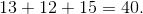Example Question #7 : Using Addition Within 100 To Solve Word Problems

At the cakewalk there arechocolate cakes,yellow cakes, andwhite cakes. How many total cakes are there?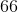Explanation:

This is an addition problem because we want to know how many total cakes are at the cakewalk all together. We can add the numbers in any order,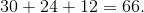Example Question #8 : Using Addition Within 100 To Solve Word Problems

At Linda’s birthday party there werepeperoni pizzas,sausage pizzas, andcheese pizzas. How many total pizzas were there?Explanation:

This is an addition problem because we want to know how many total pizzas they have. We can add the numbers in any order,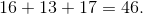Example Question #9 : Using Addition Within 100 To Solve Word Problems

There are two after-school programs at Steve’s school. One program hasstudents and the other program has. How many total students go to the after-school programs?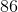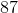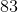Explanation:

This is an addition problem because we want to know how many total students are in the after-school programs altogether. We can either add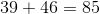or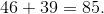Example Question #11 : Use Addition And Subtraction Within 100 To Solve One And Two Step Word Problems: Ccss.Math.Content.2.Oa.A.1

The carnival game hasdifferent sizes of bears for prizes. There aresmall bears,medium bears, andlarge bears. How many total bears does the carnival game have?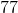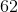Explanation:

This is an addition problem because we want to know how many total bears the carnival has altogether. We can add the numbers in any order,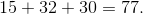Example Question #12 : Use Addition And Subtraction Within 100 To Solve One And Two Step Word Problems: Ccss.Math.Content.2.Oa.A.1

Joe spent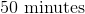cleaning his room,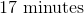cleaning his bathroom, and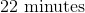helping his mom clean the kitchen. How much time did Joe spend cleaning?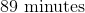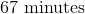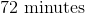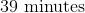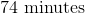This is an addition problem because we want to know how much total time Joe spent cleaning all together. We can add the numbers in any order,